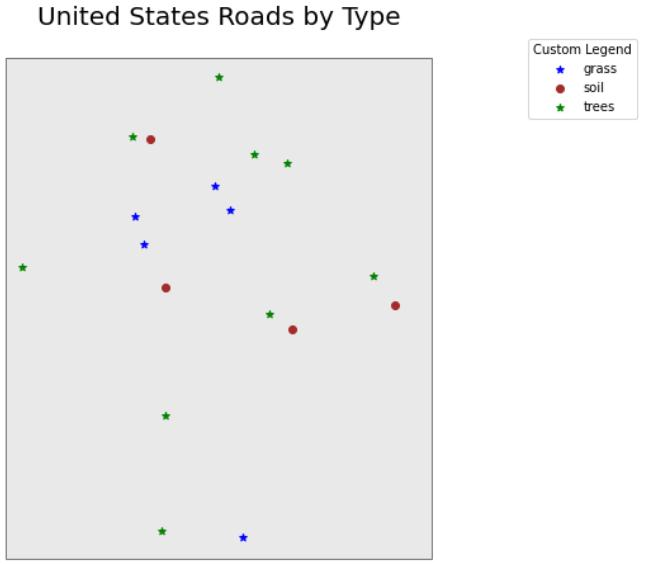# Python 中的 GIS：矢量格式空间数据简介

## Python 中的 GIS：矢量格式空间数据简介

### 关于矢量数据

• ：每个单独的点都由单个 x、y 坐标定义。矢量点文件中可以有很多点。点数据的示例包括： 采样位置、单棵树的位置或地块的位置。
• 线：线由许多（至少 2 个）连接的顶点或点组成。例如，一条道路或一条溪流可以用一条线来表示。这条线由一系列线段组成，道路或溪流中的每个“弯道”代表一个具有定义 x, y 位置的顶点。
• 多边形：多边形由 3 个或更多个相连且 “closed” 的顶点组成。因此，地块边界、湖泊、海洋和州或国家的轮廓通常由多边形表示。有时，多边形中间可能有一个洞（如环状物），这是需要注意的事情，但不是将在本教程中处理的问题。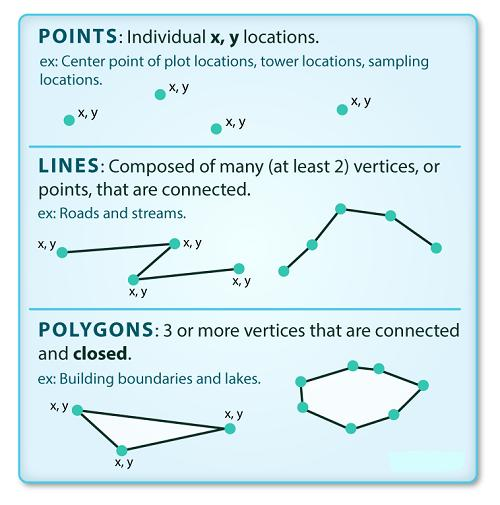### 一个数据集 - 多个文件

Shapefile 结构

• .shp：包含所有要素几何的文件。
• .shx：索引几何的文件。
• .dbf：以表格格式存储要素属性的文件。

• .prj：包含投影格式信息的文件，以及坐标系和投影信息。它是一个纯文本文件，使用众所周知的文本 (WKT) 格式描述投影。
• .sbn.sbx：作为要素空间索引的文件。
• .shp.xml：XML 格式的地理空间元数据文件（例如 ISO 19115 或 XML 格式）。

### 导入 Shapefiles

# import necessary packages
import os
import matplotlib.pyplot as plt
import geopandas as gpd
import earthpy as et

et.data.get_data("spatial-vector-lidar")
os.chdir(os.path.join(et.io.HOME, 'earth-analytics'))

Extracted output to /root/earth-analytics/data/spatial-vector-lidar/.


• 代表现场边界的多边形形状文件，
• 代表道路的线形文件，

# import shapefile using geopandas


CRS UTM 区域 18N，CRS extent 在指定单位时对于解释对象值至关重要。

### 空间数据属性

shapefile 中的每个对象都有一个或多个与之关联的属性。Shapefile 属性类似于电子表格中的字段或列。电子表格中的每一行都有一组与其关联的列，这些列描述行元素。在 shapefile 的情况下，每一行代表一个空间对象，例如，一条道路，表示为线 shapefile 中的一条线，将有一个“行”属性与之关联。这些属性可以包括描述存储在 shapefile 中对象的不同类型信息。因此，道路可能有名称、长度、车道数、限速、道路类型和其他存储的属性。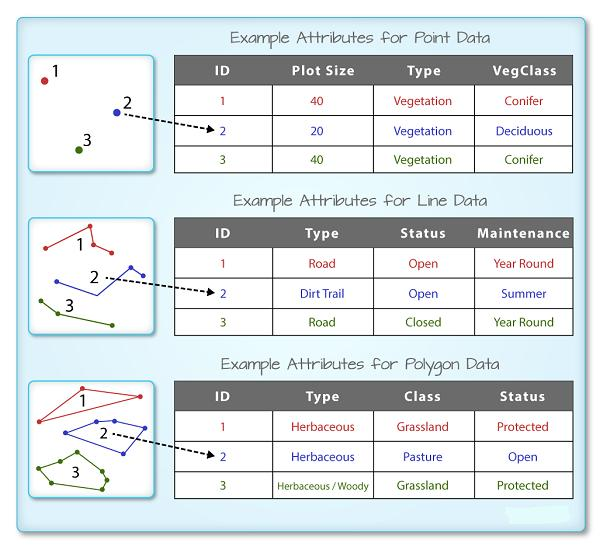R 空间对象中的每个空间特征都具有相同的一组描述或表征该特征的关联属性。属性数据存储在单独的 *.dbf 文件中。属性数据可以与电子表格进行比较。电子表格中的每一行代表空间对象中的一个要素。

GeoDataFrame 只需在控制台中输入对象名称（例如，sjer_plot_locations），即可查看与 geopandas 关联的属性表。使用的 .head(3) 函数仅显示属性表的前 3 行。请记住，函数中的数字 .head() 表示函数将返回的总行数。

# view  the top 6 lines of attribute table of data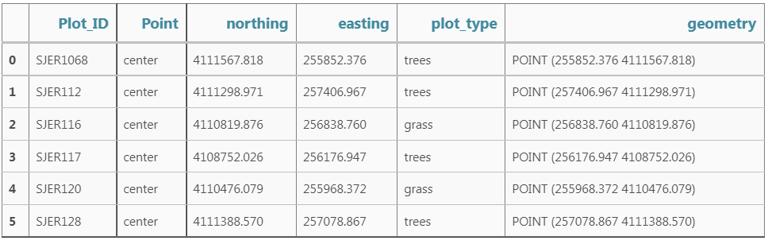• Plot_ID、Point、easting、geometry、northing、plot_type

Geopandas 数据结构

### 空间元数据

• 对象类型：导入对象的类。
• 坐标参考系统 (CRS)：数据的投影。
• 范围：shapefile的空间范围（shapefile 覆盖的地理区域）。

type(sjer_plot_locations)
## geopandas.geodataframe.GeoDataFrame

# view the spatial extent
sjer_plot_locations.total_bounds
## array([ 254738.618, 4107527.074,  258497.102, 4112167.778])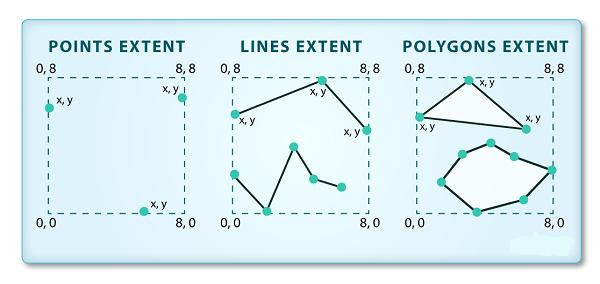shapefile 或 geopandas GeoDataFrame 的空间范围表示最北、最东南和最西的地理“边缘”或位置。因此表示空间对象的整体地理覆盖范围。

sjer_plot_locations.crs
## <Projected CRS: EPSG:32611>
Name: WGS 84 / UTM zone 11N
Axis Info [cartesian]:
- E[east]: Easting (metre)
- N[north]: Northing (metre)
Area of Use:
- name: World - N hemisphere - 120°W to 114°W - by country
- bounds: (-120.0, 0.0, -114.0, 84.0)
Coordinate Operation:
- name: UTM zone 11N
- method: Transverse Mercator
Datum: World Geodetic System 1984
- Ellipsoid: WGS 84
- Prime Meridian: Greenwich


sjer_plot_locations.geom_type
## 0     Point
1     Point
2     Point
3     Point
4     Point
5     Point
6     Point
7     Point
8     Point
9     Point
10    Point
11    Point
12    Point
13    Point
14    Point
15    Point
16    Point
17    Point
dtype: object


### Shapefile 中有多少特征？

（行，列）

sjer_plot_locations.shape
## (18, 6)


### 绘制 Shapefile

dataframe_name.plot()

# plot the data using geopandas .plot() method
fig, ax = plt.subplots(figsize = (10,10))
sjer_plot_locations.plot(ax=ax)
plt.show()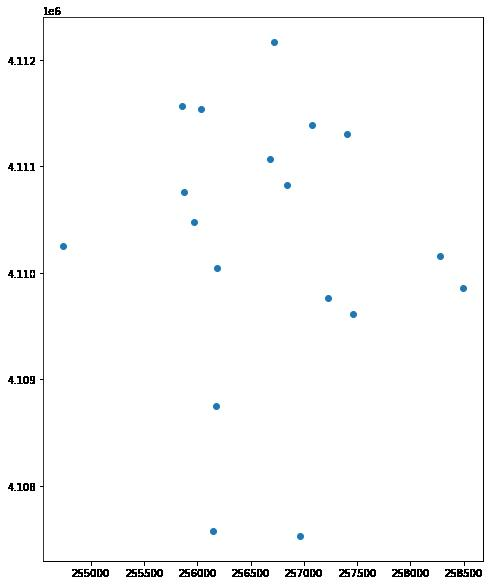• column：要使用绘制数据的属性列
• categorical=True：将绘图设置为绘制分类数据 - 在本例中为绘图类型
• 图例：添加图例
• markersize：增加或减少绘图上呈现的点或标记的大小
• cmap：设置用于绘制数据的颜色

fig, ax = plt.subplots(figsize = (10,10))

# quickly plot the data adding a legend
sjer_plot_locations.plot(column='plot_type',
categorical=True,
legend=True,
figsize=(10,6),
markersize=45,
cmap="Set2", ax=ax);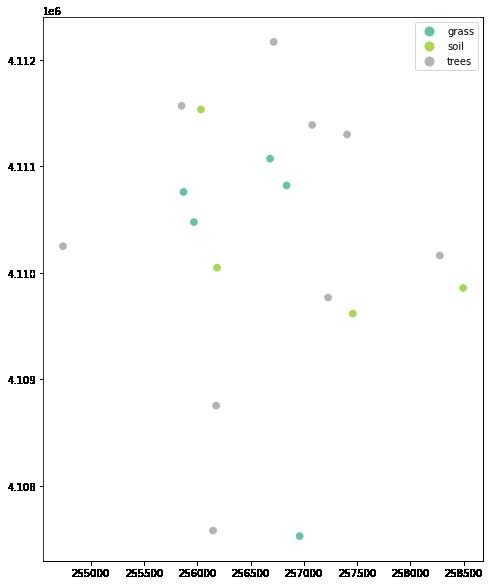# Plot the data adjusting marker size and colors
# # 'col' sets point symbol color
# quickly plot the data adding a legend
ax = sjer_plot_locations.plot(column='plot_type',
categorical=True,
legend=True,
figsize=(10,10),
markersize=45,
cmap="Set2")
# add a title to the plot
ax.set_title('SJER Plot Locations\nMadera County, CA', fontsize=16);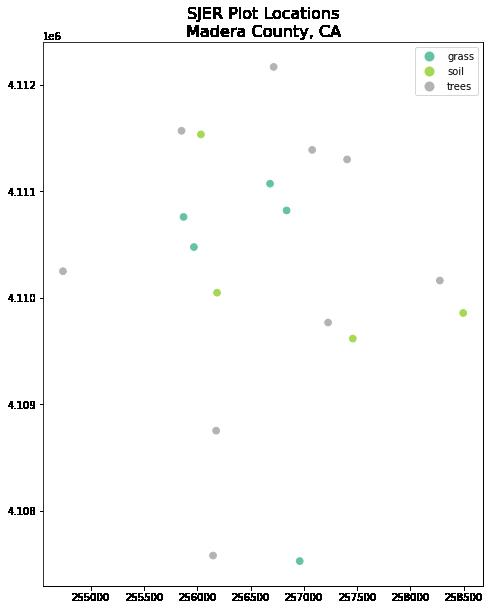fig, ax = plt.subplots(figsize = (10,10))

sjer_plot_locations.plot(column='plot_type',
categorical=True,
legend=True,
marker='*',
markersize=65,
cmap='OrRd', ax=ax)
# add a title to the plot
fontsize=16)
plt.show()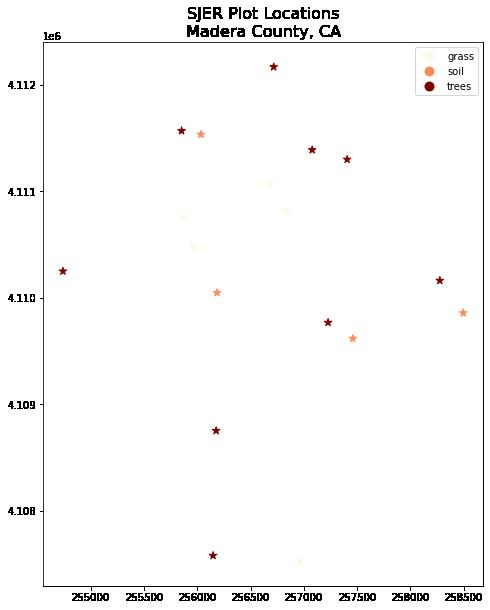ax = sjer_plot_locations.plot(figsize=(10, 10),
column='plot_type',
categorical=True,
marker='*',
markersize=65,
cmap='OrRd')
# add a title to the plot
fontsize = 16)
plt.show()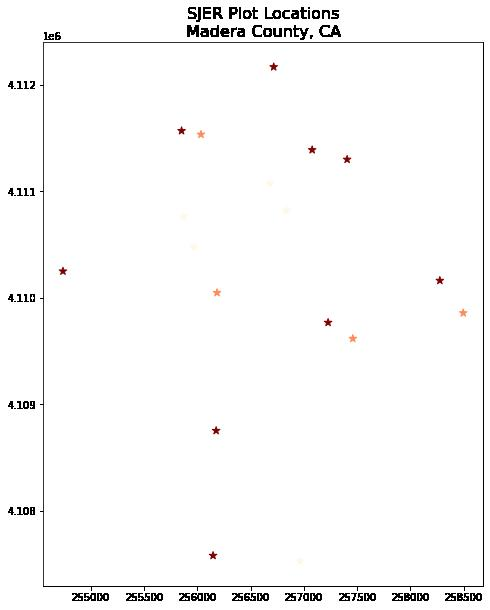### 绘制多个 Shapefiles

• ax 像上面那样定义变量，为我们的图添加标题。
• 使用 geopandas .plot()方法向图中添加尽可能多的图层。

• ax.set_axis_off()用于关闭 x 和 y 轴,
• plt.axis('equal') 用于确保 x 和 y 轴均匀间隔。
# import crop boundary

fig, ax = plt.subplots(figsize = (10, 10))

# first setup the plot using the crop_extent layer as the base layer
sjer_crop_extent.plot(color='lightgrey',
edgecolor = 'black',
ax = ax,
alpha=.5)
# then add another layer using geopandas syntax .plot, and calling the ax variable as the axis argument
sjer_plot_locations.plot(ax=ax,
column='plot_type',
categorical=True,
marker='*',
legend=True,
markersize=50,
cmap='Set2')
# add a title to the plot
ax.set_axis_off()
plt.axis('equal')
plt.show()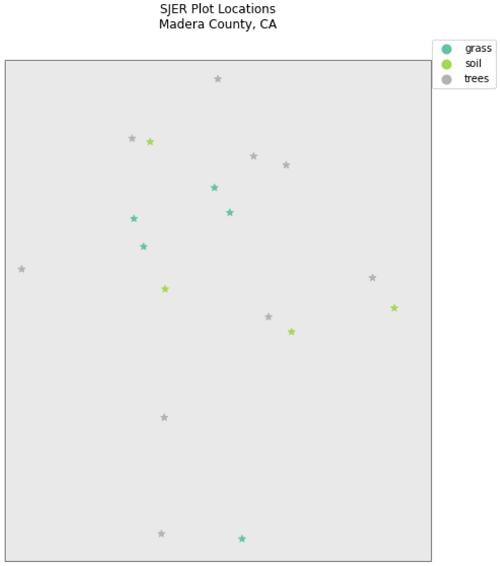### 带有 Geopandas 的自定义图例 - 可选

# make it a bit nicer using a dictionary to assign colors and line widths
plot_attrs = {'grass': ['blue', '*'],
'soil': ['brown','o'],
'trees': ['green','*']}

# plot the data
fig, ax = plt.subplots(figsize  = (12, 8))

# first setup the plot using the crop_extent layer as the base layer
sjer_crop_extent.plot(color='lightgrey',
edgecolor = 'black',
ax = ax,
alpha=.5)

for ctype, data in sjer_plot_locations.groupby('plot_type'):
data.plot(color=plot_attrs[ctype],
label = ctype,
ax = ax,
marker = plot_attrs[ctype],
)

ax.legend(title="Custom Legend")
ax.set_title("United States Roads by Type", fontsize=20)
ax.set_axis_off()
plt.axis('equal')
plt.show()